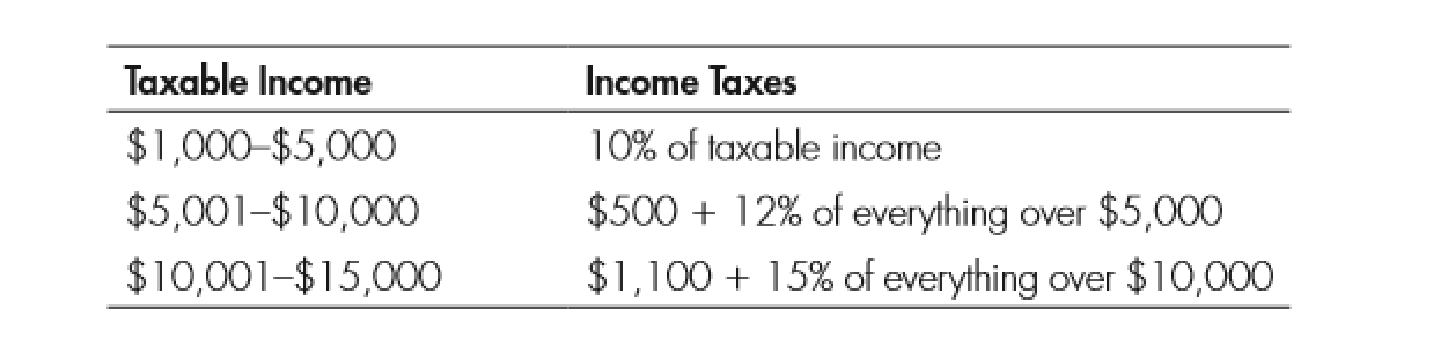Chapter 11, Problem 2WNG

Chapter
Section
Textbook Problem

Use the following table to answer questions 1–4:2. If a person’s income is $14,000, how much does she pay in taxes? To determine Identify the taxable amount. Explanation As per the given table, people who lie under the income range between$10,001 and $15,000 have to pay$1,100 plus 15% of everything over $10,000 as tax. Since the income is$14,000, 15% of $4,000 ($14,000-$10,000) is$600

Still sussing out bartleby?

Check out a sample textbook solution.

See a sample solution

The Solution to Your Study Problems

Bartleby provides explanations to thousands of textbook problems written by our experts, many with advanced degrees!

Get Started

Find more solutions based on key concepts